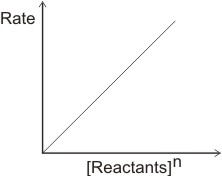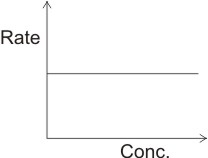## The Order of a Chemical Reaction

The rate of a chemical reaction in any instance is dependent on the concentration of the reactants. This dependence however is not always linear. I.e., rate α [reactants]n - this is known as the rate law. The exponent, n is defined as the order of the reaction.

When n is = 1, the reaction is said to be first order. When n = 2, the reaction is second order, and the reaction is third order when n = 3.

A plot of rate against [reactants]n will give a linear graph.

I.e.where n is not zero.

For the reaction A + B → C + D

If the rate is dependent on reactants A and B, then the rate law expression may be written as; rate = [A]m [B]n. The total order of the reaction is m + n, and the reaction is said to be mth order with respect to A and nth order with respect to B.

Example, if a reaction such as 2NO + O2 → 2NO2 were found experimentally to be first order with respect to oxygen and second order with respect to NO, then the rate law expression may be written as: rate α [NO]2 [O2].

The total order of the reaction is 2 + 1 = 3

Note: the order of a chemical reaction cannot be obtained from the stoichiometry of the reaction, but from a mathematical analysis of the experimental data.

First Order Reactions

A first order reaction is one in which the rate of the reaction is proportional to the first power of the concentration of the reactant which determines the rate law. Considering the hypothetical reaction:

A → products (A is the reactant which determines the rate of the reaction). Hence the rate law expression can be written as:

Rate = -d[A]/dt = k1[A]

k is called the specific rate constant.

Examples of first order reactions include: (i). Many decomposition reactions - the decomposition of hydrogen peroxide and phosphorus pentachloride

I.e. 2H2O2 → 2H2O + O2;

PCl5 → PCl3 + Cl2;

Other first order reactions are:

(i). Radioactive decay - example, Uranium → radium → lead

(ii). Hydrolysis of an ester - CH3COOC2H5 + H2O + H+ → CH3COOH + C2H5OH

Second Order Reactions

A second order reaction is one in which the rate of reaction depends either on two molecules, each having its concentration raised to a power of one or only on one molecule whose concentration is raised to a power of two.

Example, Considering that the hypothetical reaction A → B is second order kinetics, then the equation may be written as

Rate = -d[A]/dt = k2[A]2

Or, if the reaction A + B → C + D is second order, and the rate is determined by reactants A and B, then the rate equation may be written as rate = k2 [A][B]

Examples of second order reactions: some chemical combinations like that of hydrogen and iodine to form hydrogen iodide; some polymerization reactions, example, polymerization of ethene; hydrolysis of bromoethane - C2H5Br + NaOH → C2H5OH + NaBr; some thermal dissociations, example, of hydrogen iodide, nitrogen(IV) oxide, ozone, formaldehyde, cyclobutane and acetaldehyde.

Zero Order Reactions

A zero order reaction is one in which the rate of the reaction does not depend on the reactants nor on the products. It is very rare to have reactions of this type. The rate equation is written as rate α [reactant]o i.e.,

Rate = dx/dt = k

By plotting rate against concentration of reactants, the graph below is derived.This shows that the rate does not depend on concentration of reactants or products. An example of a zero order reaction is the combination of hydrogen and chlorine over water at constant pressure.

 Like This Post? Please Share!!!!

Copyright , All Rights Reserved Free Chemistry Online | About Us | Usage of Content | Total Disclosures | Privacy Policy